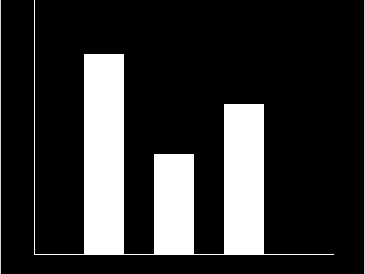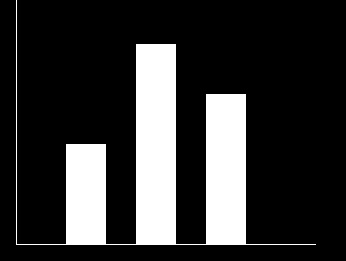# bar() function in C graphics

The header file graphics.h contains bar() function which is used to draw a 2-dimensional, rectangular filled in bar.
Syntax :

```void bar(int left, int top, int right, int bottom);

where,
left specifies the X-coordinate of top left corner,
top specifies the Y-coordinate of top left corner,
right specifies the X-coordinate of right bottom corner,
bottom specifies the Y-coordinate of right bottom corner.
```

Examples :

```Input : left = 150, top = 150,
right = 190, bottom = 350

left = 220, top = 250,
right = 260, bottom = 350

left = 290, top = 200,
right = 330, bottom = 350
Output :Input : left = 150, top = 250,
right = 190, bottom = 350

left = 220, top = 150,
right = 260, bottom = 350

left = 290, top = 200,
right = 330, bottom = 350
Output :```

## Recommended: Please try your approach on {IDE} first, before moving on to the solution.

Below is the implementation of bar() function.

 `// C implementation of bar() function ` `#include ` ` `  `// driver code ` `int` `main() ` `{ ` `    ``// gm is Graphics mode which is ` `    ``// a computer display mode that ` `    ``// generates image using pixels. ` `    ``// DETECT is a macro defined in ` `    ``// "graphics.h" header file ` `    ``int` `gd = DETECT, gm; ` ` `  `    ``// initgraph initializes the ` `    ``// graphics system by loading a ` `    ``// graphics driver from disk ` `    ``initgraph(&gd, &gm, ``""``); ` ` `  `    ``// location of sides ` `    ``int` `left, top, right, bottom; ` ` `  `    ``// left, top, right, bottom denotes ` `    ``// location of rectangular bar ` `    ``bar(left = 150, top = 150,  ` `    ``right = 190, bottom = 350); ` ` `  `    ``bar(left = 220, top = 250, ` `    ``right = 260, bottom = 350); ` ` `  `    ``bar(left = 290, top = 200, ` `    ``right = 330, bottom = 350); ` ` `  `    ``// y axis line ` `    ``line(100, 50, 100, 350); ` ` `  `    ``// x axis line ` `    ``line(100, 350, 400, 350); ` ` `  `    ``getch(); ` `     `  `    ``// closegraph function closes the ` `    ``// graphics mode and deallocates ` `    ``// all memory allocated by ` `    ``// graphics system . ` `    ``closegraph(); ` `     `  `    ``return` `0; ` `} `

Output:

``````

My Personal Notes arrow_drop_upCheck out this Author's contributed articles.

If you like GeeksforGeeks and would like to contribute, you can also write an article using contribute.geeksforgeeks.org or mail your article to contribute@geeksforgeeks.org. See your article appearing on the GeeksforGeeks main page and help other Geeks.

Please Improve this article if you find anything incorrect by clicking on the "Improve Article" button below.

Article Tags :
Practice Tags :

Be the First to upvote.

Please write to us at contribute@geeksforgeeks.org to report any issue with the above content.